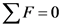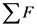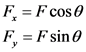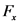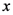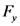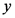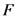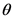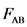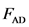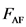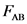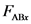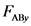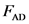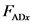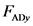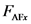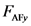# What is the magnitude of the force provided by the third rope. in terms of θ?

A boat owner pulls her boat into the dock shown, where
thereare six capstans to which to tie the boat. She has three
ropes. Shecan tie the boat from the boat’s center (A) to any of the
capstans(B through G) along the dotted arrows shown.
Suppose the owner has tied three ropes: one rope runs to A from
B,another to A from D, and a final rope from A to F. The ropes
aretied such that
.The following notation is used in
thisproblem: When a question refers to, for example, , this
quantity is taken to mean the force acting on theboat due to the
rope running to A from B, while is the
magnitude of that force.The following notation is used in
thisproblem: When a question refers to, for example, , this
quantity is taken to mean the force acting on theboat due to the
rope running to A from B, while is the
magnitude of that force. What is the
magnitude of the force provided by thethird rope, in terms of
?
A. FABCos(θ)
B. 2FABCos(θ)
C. 2FABSin(θ)
D.FABSin(θ)
I think its C. Am i right?What is the
magnitude of the force provided by thethird rope, in terms of
? What is the
magnitude of the force provided by thethird rope, in terms of
? A. FABCos(θ) AB B. 2FABCos(θ) AB C. 2FABSin(θ) AB D.FABSin(θ) AB I think its C. Am i right?

General guidance

Concepts and reason

The concepts required to solve this problem are equilibrium force condition and vector components.
Initially, use the component equation of a force to resolve the forces along the axes. Then, determine the net force along the horizontal direction. Finally, use the equilibrium force condition to solve for the magnitude of the force by third rope.

Fundamentals

The equilibrium force condition gives that the sum of all the forces on an object is zero when it is not accelerating. This is expressed as,

Here, is sum of all the forces on the object.
The components of a force are as follows:

Here, is the component of the force, is the component of the force, is the magnitude of force, and is the angle between the axis and force in counterclockwise direction.
Sign Convention is as follows:
Take all the forces in upward or towards right as positive and all the forces in downward or towards left as negative.

Step-by-step

Step 1 of 2

Draw the diagram of the boat and ropes showing the direction of the forces. ,, and are the forces acting on the boat due to the rope running to A from B, to A from D, and to A from F. The components of the force along and components are and . The components of the force along and components are and . The components of the force along and components are and .

Use the component equation of a force and substitute for , and for in the equation .

Use the component equation of a force and substitute for , and for in the equation .

In the above diagram, a boat owner pulls her boat into the dock there are six bollards to which to tie the boat. Owner has three ropes and can tie the boat from the boat’s center A to any of the bollards. One rope runs to A from B, another to A from D, and a final rope from A to F.

The component of the force along the horizontal direction is not given as, . The component of the force along the horizontal direction is given as, . Here, is the angle between the force and the horizontal component of the force.

Use the equilibrium force condition to solve for the magnitude of force provided by third rope.

Step 2 of 2

Use the equilibrium force condition along axis.

Three forces act on the boat while it is stationary, and towards left so negative and towards right so positive. Substitute for in the above equation .

Substitute for , and for in the above equation.

Since, substitute for in the above equation.

The magnitude of force provided by the third rope in terms of is . So, the options are not correct.

The magnitude of force provided by the third rope in terms of is .

Three forces act on the boat while it is stationary, and towards left so negative and towards right so positive. So, the net force acting on the boat along the horizontal direction is,

The equilibrium force condition gives that the sum of all the forces on an object is zero when it is not accelerating is zero. As the boat is not accelerating along the horizontal direction. The net force along the horizontal direction is zero. That is,

The net force along the horizontal direction is not given as, . As the forces and are acting towards and towards right, so the net force along the horizontal direction is given as,

The magnitude of force provided by the third rope in terms of is .

The magnitude of force provided by the third rope in terms of is .

ΣF = 0
F = F cos F, = F sin
Boat Figure 1: The boat and rope diagram with all the forces and its components.
F = F cos
Fare = Fab cose
F = F cos
Fadx = Fan cos
F = F sin
F = F cos
ΣF = 0
FF – FABE – FADE
ΣF = 0
Far – FABr – Fadx = 0 Far = FABr +FADE
FAB cos
Fap cos
Far = FAB cosO+Fap cos
FFD
Far = FAB cos 0 + FAB cos = 2F cos
2FAB cos
FAB cos 0, 2F 3 sin 0 and Fall sin
F=FF-FAB – Fax
ΣF = 0 Fx – Fikr – Fxdx = 0
EF = Fxp+Fxxx + FADE
F=FF-FAB – Fax
2FAB cos
2FAB cos
We were unable to transcribe this image